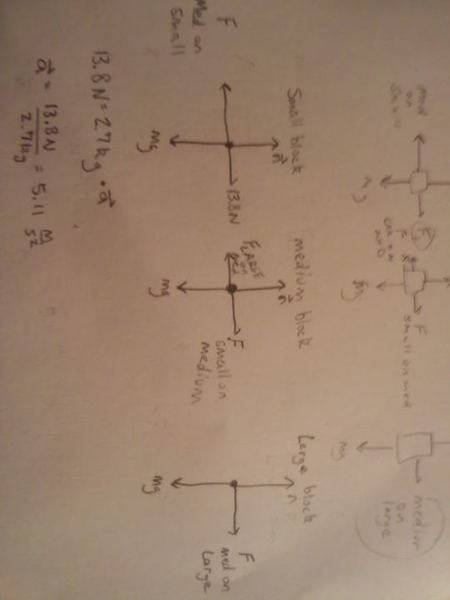# 3 blocks on a frictionless surface

## Homework Statement

Blocks with masses of 0.54 kg, 2.16 kg, and 2.87 kg are lined up in a row on a frictionless table. All three are pushed forward by a 13.8 N force applied to the 1 kg block. How much force does the 2.16 kg block exert on the 2.87 kg block?

Fnet=ma

## The Attempt at a SolutionI thought all I had to do was calculate the acceleration and then multiply by the mass of the second block?

There is a second part of the problem that I got right.....
How much force does the 2.16 kg block exert on the 0.54 kg block?
I found the acceleration which is 13.8/5.57=2.48 m/s^2 then i took that and mutliplied it by .54 kg that gave me N then I subtracted that N from the orginal 13.8.....but it doesn't work for the first part why?

PhanthomJay
Homework Helper
Gold Member
Blocks with masses of 0.54 kg, 2.16 kg, and 2.87 kg are lined up in a row on a frictionless table. All three are pushed forward by a 13.8 N force applied to the 1 kg block. How much force does the 2.16 kg block exert on the 2.87 kg block?

I thought all I had to do was calculate the acceleration and then multiply by the mass of the second block?
No, this will give you the net force acting on the 2nd block, not the force of block 2 on block 3. Instead, once you calculate the acceleration, you must draw a Free Body Diagram (FBD) which isolates the last (2.87 kg) block, identify the forces acting on that block, and apply Newton's laws to that block to solve for the force of block 2 on block 3.

There is a second part of the problem that I got right.....
How much force does the 2.16 kg block exert on the 0.54 kg block?
I found the acceleration which is 13.8/5.57=2.48 m/s^2
Correct.
then i took that and mutliplied it by .54 kg that gave me N
no it didn't, it gave you the net force acting on block 1
then I subtracted that N from the orginal 13.8.....but it doesn't work for the first part why?
you mean you subtracted the net force from the 13.8 to get the force of block 2 on block 1.

Yeah I meant I subtracted the net force from 13.8. I got the first one but not the second one.....hmmmm i think I will meet with my professor and ask him what i should have done. thank you guys for your help.